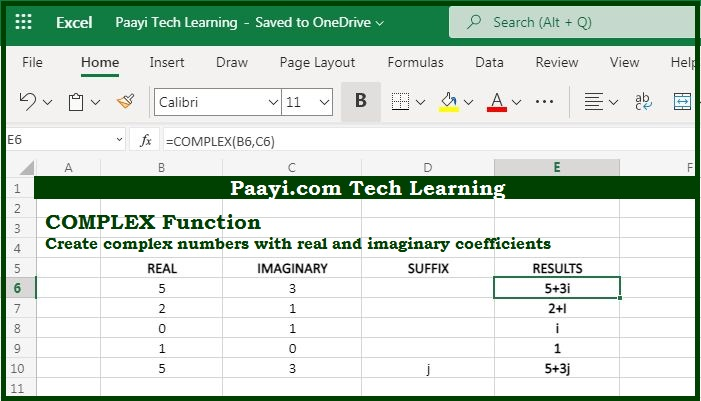# Learn How to Use Microsoft Excel COMPLEX Function

Written by | 0 Comments | 725 Views

In this article, you will learn how to use the Microsoft Excel COMPLEX function and its prime function in Microsoft Excel. You will also get to know the Microsoft Excel COMPLEX function return value and syntax with the help of some examples.

Microsoft Excel COMPLEX Function

The prime function of the Microsoft Excel COMPLEX function is to covert coefficients to complex numbers. So, with the help of the COMPLEX function, you can able to create complex numbers with the provided real and imaginary coefficients. The COMPLEX function returns the results as - "x + yi" or "x + yj."

Return Value of COMPLEX Function

The return value will be the complex number as a text.

Syntax of COMPLEX Function

=COMPLEX(real-num, i-num, [suffix])

Where the argument:

real-num: It is the real number.

i-num: It is the imaginary number.

suffix: It is the suffix either "i" or "j" (optional).

## How to Use Microsoft Excel COMPLEX Function?As we know that complex numbers are having two parts - a real number and an imaginary number. And you can convert the real and imaginary coefficients into a complex number in the form "x + yi" or "x + yj" with the help of the COMPLEX function. Here "x" represents the real number and "y" represents the imaginary number.

You should remember that if the suffix omitted, the suffix defaults to "i". The suffix must be in the lowercase "i" and "j" and in case the real-num or i-num are non-numeric COMPLEX function returns the #VALUE! error.